+ +

# Huygens (principle of)

The Huygens principle concerns the propagation of light in the form of transmitted wavelets and re issued successively. It's the astronomer and Dutch mathematician Christian Huygens (1629-1695), that must be the first theorization of the wave front. It was inspired by the properties of mechanical waves, which he pulled the principle of the waves 'envelopes', which since door its name.

### Mechanical analogy

By observing the fact that a ripple produced on the surface of the water after the fall of a rock is spread by close in close action, he understood that any point of this reaches surface by the wave should its direct neighbouring points action and movement not to the action of the primary source (place of the fall of the stone). In this theory, any point reached by the wave then acts as a secondary source which, in turn, radiates a Wavelet in direction of the neighbourhood. As a result, the status of the vibration of the surface of the water at a given time allows to predict its future position: it is not necessarily need to know the characteristics of the initial pulse.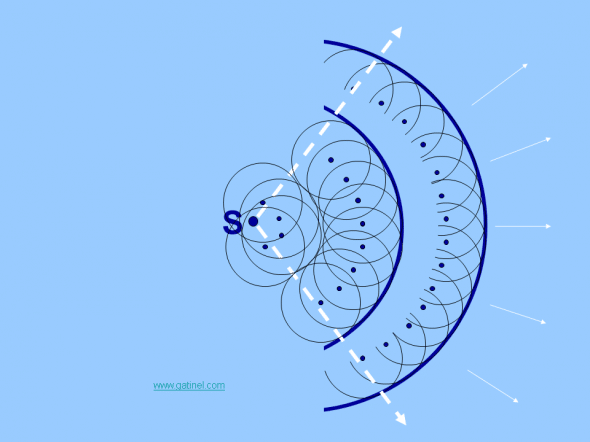The Huygens principle is based on restitution by adjacent points of the impulse of the original source in the form of wavelets.

### Bright Wavelet

Huygens transposed these findings to light and applied that a light source emitted successive impulses, giving rise to a disturbance located on a surface called "wave front", which was spreading in a specific environment ("ether"). At the time of Huygens, the notion of electromagnetic field was not known, and light waves should necessarily have a 'support' for this spread. Huygens designed the process of spread of light Wavelet and mechanical vibrations in an elastic medium.

If a wave front has a certain position at a given moment t, his later position is determined as the envelope of wavelet issued at this time t by points distributed secondary sources its original position. In a homogeneous medium, the waves retain their plan or spherical character.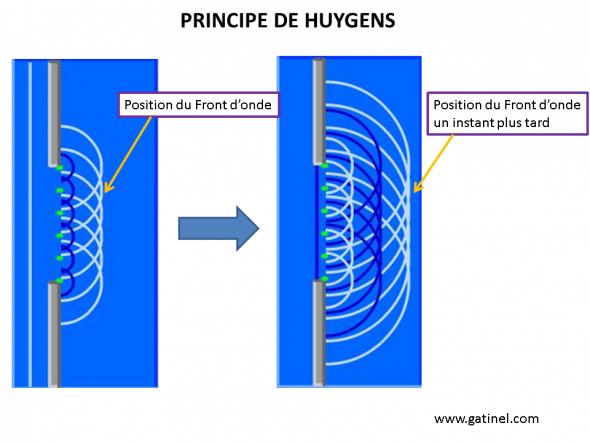The propagation of a wavefront at a later time can be inferred from the position of the wavelet to a previous time.

### Light propagation and interference

The principle of Huygens has been completed and mathematically formulated by Fresnel at the beginning of the 19th century, including the concept of interference; "this concept explained that there was no wave re issued"backward"when" light vibration "contiguous sources. Indeed, in a dense homogeneous medium, there is little or no light scattered sideways or backward. Earth's atmosphere contains millions of molecules in a cube which stops it would be equal to a visible light wavelength (ex: 500 nm). This is related to the size of the molecules, the nanometer, while the visible light wave is of the order of a micron.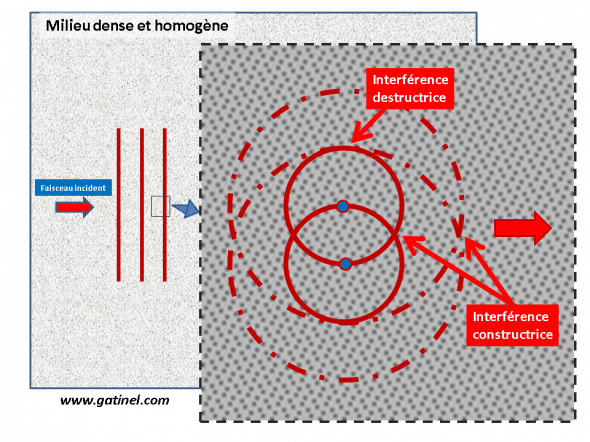Consider a plane wave propagates in a medium dense and homogeneous, composed of many contiguous atoms, represented here schematically by 'points. At the level of atoms, the light is absorbed and reemitted by electrons. This phenomenon is responsible for changes in the "phase" of the wavefront, which can then appear as slowed in the propagation media (the index of refraction is proportional to the slowdown of the transmitted wave). Perpendicular to the propagation direction, we can form many pairs of atoms separated by half a wavelength (atoms are about a thousand times smaller than the relevant visible wavelength). Given the principle of Huygens, each atom reemits the incident wave in all directions. Thus, in a perpendicular direction from the main direction the light spread, there is destructive interference for each of these pairs of atoms, which explains the absence of lateral diffusion of the light. Given the principle of conservation of energy, there should be constructive interference in all directions. In a sparse environment (ex: upper atmosphere), because of the scarcity of the atoms and molecules, the phenomena of interference disappear. Then, there are random "encounters" between light and atoms, of lateral reemissions, which can show that they are proportional to the (power 4) frequency of the light wave. Blue (high frequency) is more scattered than the red, and this explains the color of the sky: our eyes perceive a bluish hue because that shorter wavelength is more scattered laterally, so toward the ground.

### Huygens principle and law of Snell-Desmaps

In examining the consequences of the Huygens principle, it is important to realize that the emitted wave fronts consecutively are separated by equal time intervals. This property allows to prove the law of refraction of Snell (known as Snell-Desmaps in France). Refraction occurs when the wave front meets a different environment and that its rate of spread is reduced.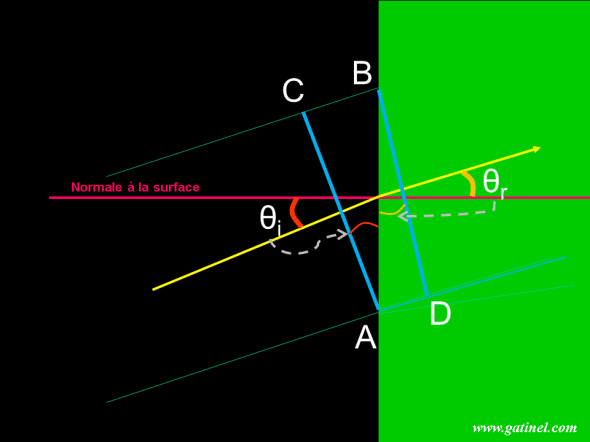In the middle of the left, the Wavefront moves at speed VI. On the right, its speed (VR) decreases. The Wavefront forms an θi angle with normal at the surface, and is deflated from an angle θr after refraction. According to the principle of Huygens, the time set by the light to go from C to B is the same as the one to go from A to D. This time is equal to the distance divided by the speed, and one can ask: CB/VI = AD/VR according to the geometry of the figure, and observing that θi is equal to the angle (CAB) and that θr is equal to the angle (ABD) can be expressed that the distance CB = AB sine (θi) and the distance AD = AB sine (θr). The first equation becomes AB sine (θi)/VI = AB sine (θr)/VR. It is possible to simplify by AB and multiply by a constant c (speed of light in the vacuum) and one obtains: sine (θi) c/VI = sine (θr) c/VR. The ratio between the velocity of light in the vacuum and the light in the medium considered is equal to the refractive index of this medium: NI = c/VI and Nr = c/VR finally: NI sine (θi) = Nr sine (θr)

Snell's law to calculate the trace of a refracted Ray, from the angle of the incident angle with the normal to the surface and the value of the indices of refraction of each community.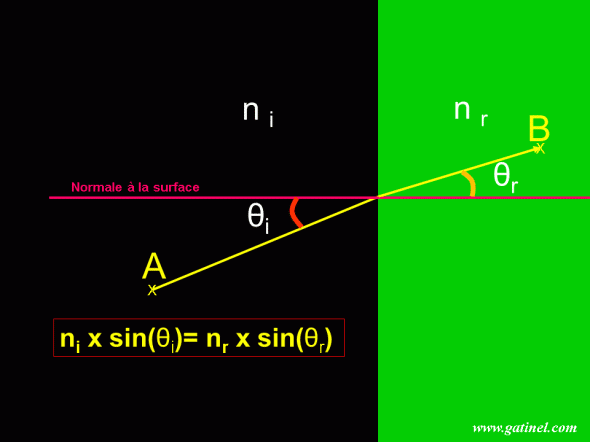The law of Snell-Desmaps allows to calculate the path of light rays through refractive surfaces. This law is a pillar of geometrical optics. It is consistent with the Fermat principle, which States that between two points, the light takes the path of minimum (and no minimum distance).

### Principle of Huygens and Wavefront

Thus, the very general principle of Huygens was be interpreted in the context of undulatory theory of light (electromagnetic waves). Suppose a light monochromatic (S) in a homogeneous medium isotropic. It emits light waves, which propagate along rays in all directions: in each point of the space close to the source earned by the light wave, the State of the electromagnetic field oscillates (at the frequency of the source), between a minimum value and a maximum value. At some point, all points located at a similar distance from the source are in the same State (they are "in phase").

When the source is placed in an isotropic medium, the envelope of the points located at the same distance from the source shape a spherical space (and circular in plan). The Wavefront is spherical, and because the waves move away from the source, it is divergent. As you move away from the source, the radius of curvature increases (the same portion of wave front is more flat). If one is far enough away from the source, an instrument of observation may collect a portion of the wave surface whose curvature can be considered to be zero (flat).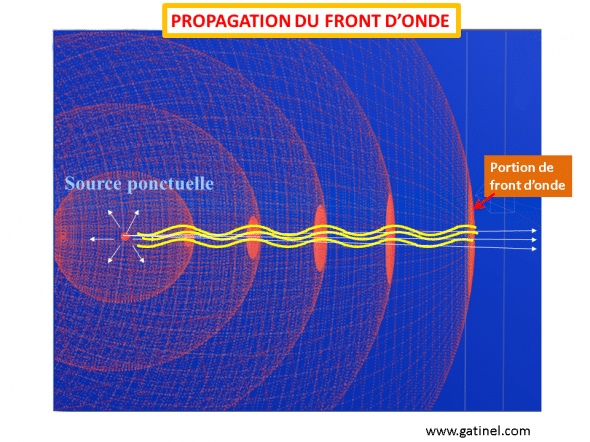The original source of light propagates a wave front that is locally perpendicular to the rays of light ('normal congruence' property). Rays from a point source are perpendicular to the sphere centered on the source. Distance from the source, a portion of wave front can be regarded as 'flat '.

The wave front is the envelope that connects these points "in phase". flat when the rays spread parallel, it is spherical and expansion as part of a wave front differ. She is also spherical but tends to be collapse to a central point in Wavefront case converge.

### 2 responses to "Huygens (principle of)"

1.Nicolas Rouher says:

In the fifth line of the commentary to the Snell-Descartes law scheme, Theta I should not be replaced by Theta r in the phrase "and theta I is equal to the angle (ADB)"?
Friendships

2.Dr. Damien Gatinel says:

Right, thanks for the careful reading, the typo is corrected!